Home » Polyhedra » Prisms

# Volume of Prisms

Prisms, are a subcategory of polyhedra. These are geometric solids which have identical ends, these ends are also considered bases (yes, two bases). All of their faces are flat and if you slice a cross section along any part of the prism, the cross section is always the same. All of prisms faces are flat and have no curved sides. Therefore the cylinder is not a prism, since it has a curved base.

The formula to get the volume of a prisms is always the same, and is calculated by multiplying the base of the prism by its length.

Below you can select the three-dimensional geometric solid of your interest, inside you will find a description, the formula for the 3D geometric solid a volume calculator so you can calculate the volume automatically.

## Geometric shapes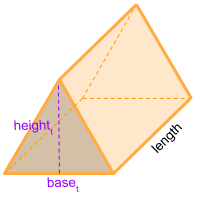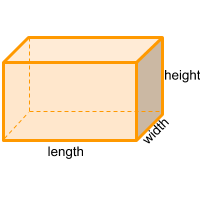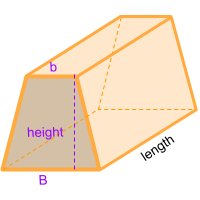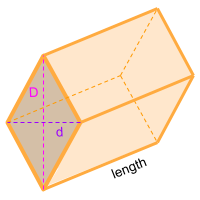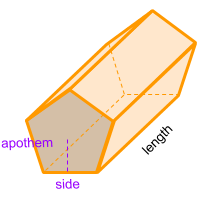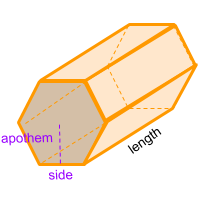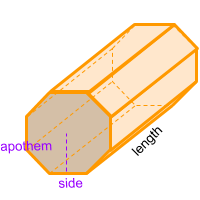Share